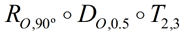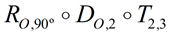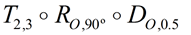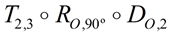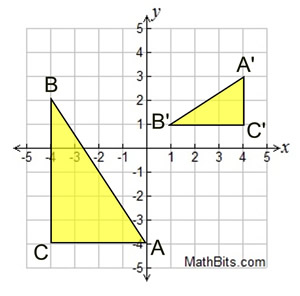Compositions of Transformations Terms of Use    Contact Person: Donna RobertsDirections: Grab paper (graph paper) and pencil. Rotations are centered at the origin unless otherwise stated.

1.
Starting with point A (-4, -5), which single translation will produce the same image as the following sequence of translations:
(x, y) → (x - 1, y + 5), followed by
(x, y) → (x + 4, y + 2), followed by
(x, y) → (x - 5, y - 7) ?
Choose:
 (x, y) → (x - 6, y - 5) (x, y) → (x - 2, y) (x, y) → (x - 1, y + 2) (x, y) → (x - 5, y)2.
Write a rule for the composition of a reflection in the x-axis following a translation of 7 units to the left and 3 units up.
Choose: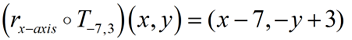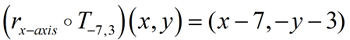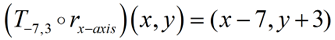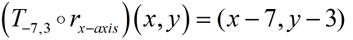3. Given rectangle MULE: M(1,2), U(1,5), L(8,5), E(8,2) a) Graph and label the following composition: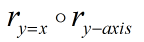b) What single transformation accomplishes      these same transformations?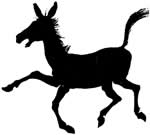4.
Triangle ABC has vertices A(-1,1), B(2,3) and C(-1,-4). Which composition of transformations will move vertex B to the point (-2,5)?
Choose: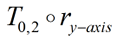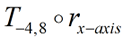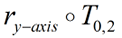All of these compositions.5 Given the graph at the right with B(-6,1), U(-3,7), G(-2,1) and B'(0,-6), U'(6,-3), G'(0,-2). Describe a sequence of transformations that was used to carry ΔBUG onto ΔB'U'G'.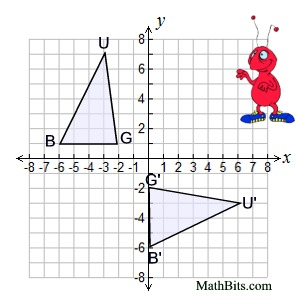6.
A double reflection occurs on the point S(-4,3) over the line x = -2, followed by the line x = 8. What is the distance between the pre-image and the image of point S?
Choose:
 8 units 10 units 12 units 20 units7 You are a contestant on the TV show "Whodunit". You are searching for a key to unlock a "Safe Card" that will save you from being killed on this week's episode. The writer for this week's show is a mathematician! Your clues relate to a coordinate map. What are the coordinates for the location of the key? Here are the three clues you have found: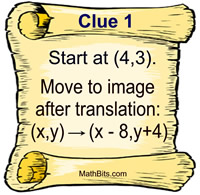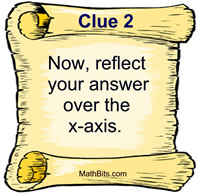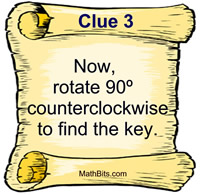8.
ΔA''B''C'' is a glide reflection of ΔABC, as shown in the graph at the right. Which statement represents the glide reflection in this situation?

Choose: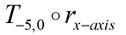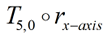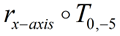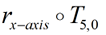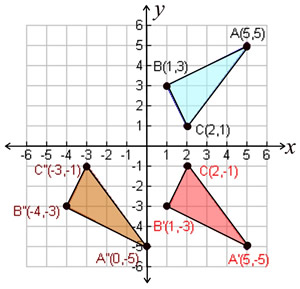9.
Point A is mapped to point A' (-1,4) after a translation of (x, y) → (x - 4, y + 1) following a reflection over y = 4.
What are the coordinates of A?
Choose:
 (3,3) (3,5) (1,3) (3,-1)10.
Which composition of transformations will map ΔABC onto ΔA'B'C' ?
Dilations and rotations are centered at the origin.
Choose: